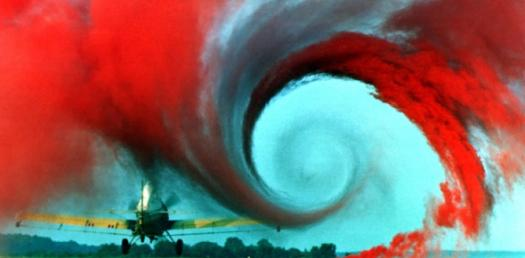# Fluid Mechanics And Hydraulics

6 Questions | Total Attempts: 408SettingsCivil Engineering

• 1.
The displacement thickness of a boundary layer is
• A.

The distance to the point where (v/V) = 0.99

• B.

The distance where the velocity 'v' is equal to the shear velocity V*, that is, where v=V*

• C.

The distance by which the main flow is to be shifted from the boundary to maintain the continuity equation

• D.

One half the actual thickness of the boundary layer

• 2.
The correct relationship among displacement thickness d, momentum thickness m and energy thickness e is
• A.

D>m>e

• B.

D>e>m

• C.

E>m>d

• D.

E>d>m

• 3.
Drag force is a function of
• A.

Projected area of the body

• B.

Mass density of the fluid

• C.

Velocity of the body

• D.

The correct answer is A, B and C

• 4.
Apart form inertia force, which of the following forces is most important in motion of submarines under water?
• A.

Viscous force

• B.

Gravity force

• C.

Compressive force

• D.

Surface tension force

• 5.
A spillway model constructed to a scale of 1:4 gives a discharge of 5 m3/s.   The discharge from the prototype would be
• A.

160 m3/s

• B.

640 m3/s

• C.

10 m3/s

• D.

20 m3/s

• 6.
A river model is made to a length scale ratio of 1/100 and depth scale ratio of 1/16.  A peak discharge of 25,600 m3/s in the river will be simulated in the model with a discharge of
• A.

10 cumec.

• B.

4 cumec.

• C.

1.6 cumec.

• D.

0.64 cumec.

Related TopicsBack to top